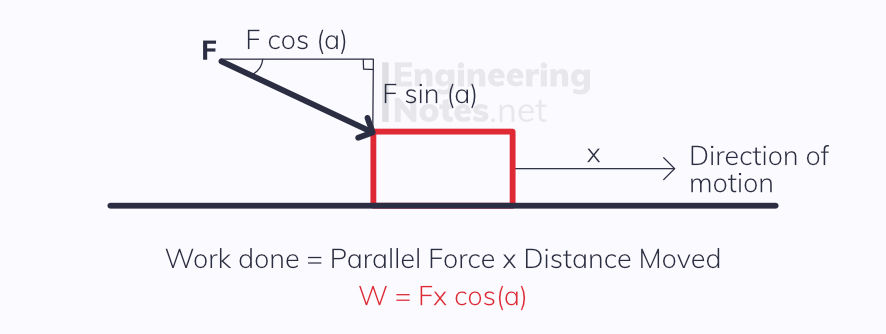top of page

#### Notes by Category University Engineering

Rate these notesNot a fanNot so goodGoodVery goodBrillRate these notes

# Work, Energy & Power

Work done is the amount of energy transferred from one form to another when a force causes movement.

The units of work are Joules, J
W = Fx Work Done = Force × distance moved (in the same direction as the force)When the force is not in the same direction as the movement of the object, you need to work out the correct component force using trigonometry.

You must remember to do this!

## Energy

One of the fundamental laws of physics is the idea that energy cannot be created. This is known as the Principle of Conservation of Energy and states:

The total energy in a closed system always remains constant - energy can never be created or destroyed, it can only ever be transferred from one form to another.

### Some of these forms include:

• Kinetic

• Gravitational Potential

• Elastic Potential

• Electrical Potential

• Nuclear

• Internal (heat/thermal)

### Kinetic Energy

All moving objects have kinetic energy:

KE = 1/2 m v^2 Kinetic Energy = 1/2 x mass x velocity squared

This equation can be derived easily from work done and SUVAT:### Gravitational Potential Energy

Gravitational Potential Energy is the energy of an object due to its positioning in a gravitational field. The greater the height, the greater the GPE.

GPE gain is work done in moving an object:

GPE = mgh

This can be derived as such:

W = Fh → F = ma = mg → GPE = mgh (g is the acceleration due to free fall, 9.81 m/s^2)

Because all energy is conserved, falling objects increase in kinetic energy as they decrease in GPE.

## Power

Power is the rate at which work is done:

P = W/t power = work done / time taken

This can also be written as:

P = Fv power = force × velocity

A derivation of this is:

P = W/t → P = Fx/t → P = Fv (Because v = x/t)

The units of power are Watts, W, or Joules per second, J/s

### Efficiency

Efficiency is the proportion of input energy that is transferred into useful output energy - for example in a motor, it is the portion of input energy that comes out as kinetic energy, rather than thermal or sound energy.

Efficiency can be calculated as a percentage:

100 x useful output energy / total input energy

See All